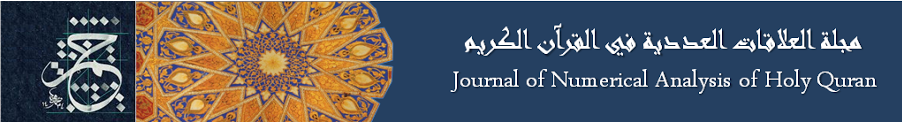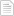### Special numbers of Quran

#### الأربعاء، 22 يوليو 2015 - 0:36The Quran Book has 114 chapters. So N=114 Pivot chapter (Al-7adeed) = N/2 = 57 The pivot chapter is a multiplication of two prime numbers P1*P2=3*19 Number of Pivot chapter verses is prime number P3 = median (1..N/2) = 29 The product of P1*P2*P3 = 3*19*29 = 1653 has the property where the 16th prime = 53أضف تعليقك
##### رجاء ضرورة الإلتزام بعدم إضافة أي تعليق يمس أو يسيء للأديان أو المعتقدات أو المقدسات, كما نرجو ألا يتضمن التعليق السباب أو أي ألفاظ تخدش الحياء والذوق العام

 الأكثر قراءةولما بلغ اشده.النحل.The 17 Suras Ending with the Letter Alef.تعيين ليلة القدر من سورة الدخان.النمل.Silver in Surat AlInsan.Surat Alqamar points to Surat ArRahman.A note about 7^n + 29^n + 139^n.Sura Alfati7a, Alfalaq and Annas all use 21 Letters of the Alphabet.لطائف للرقم 7 في سورة الفاتحة.
الموقع الرسمي حم © 2015, كل الحقوق محفوظة |